Next: Rational expressions Up: Heuristic equivalence testing and Previous: Heuristic equivalence testing and

# Modular mappings

For this heuristic we will also work with an evaluation mapping, this time over the integers mod n. This makes the computation time proportional to the expression size. Unknowns are assigned arbitrary random values in Zn. As a result, every expression is mapped onto an integer. If the expression is identically 0, any evaluation for its variables and for any n, it will evaluate to 0. So a non-zero result is proof that the expression is not identically 0. It is important that we insist on computing in time proportional to the expression size, as it is represented, and not with other measures, like degree of the polynomial, expanded size, etc. These other measures could be exponential in the size of the expression. For example consider the polynomial

((( ... (x+1)+2)2 + 3)2 + 4)2 ... +k)2

This is a dense polynomial with degree 2k but the expression is only of size O(k).

The mapping of a mathematical expression to an integer mod n will be called signature, and denoted by Sn(e). A signature is a many-to-one function. A signature function Sn(x) must distribute over arithmetic operations, that is: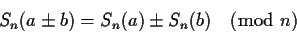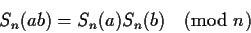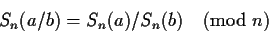This heuristic has an obvious parameter, which is n which can be set to any arbitrary value. We are going to use this feature quite extensively for two purposes. First we will select n so that all the computations are possible (or are simpler). Secondly, we will select different values of n, so that we obtain many independent results. By running this heuristic for different values of n we can exponentially reduce the probability of making a mistake.

For example, suppose that we want to test whether x10-1+(1-x5)(x5+1)is identically 0. We will use n=7 and we will map our only unknown to a random value, e.g. S(x)=2. The expression becomes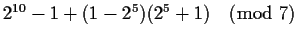. Notice that the exponentiations are done mod 7 and using binary powering and hence do not require time or size proportional to the exponent, but to the logarithm of the exponent. Since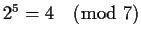and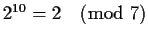the above becomes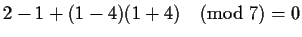. The heuristic answer is true.

Obviously this procedure can make mistakes. x-2 will also map to 0 under the same mapping. For polynomial expressions, it is quite easy to derive a bound on the number of mistakes the heuristic will make. If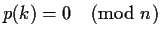, then (x-k) is a factor of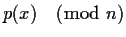. The factored polynomial can have at most d different linear factors, where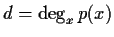. There are at most d values out of n where we can give the wrong answer. Hence for a polynomial we can bound the probability of a mistake by d/n.

Note: Computing the degree of an expression may be as difficult as zero equivalence. It is enough to have an upper bound on the degree. An easy and tight bound on the degree is 2q, where q is the number of multiplications (or divisions) used to compute the polynomial. The bound is tight since the polynomial x2q-1 can be computed with q multiplications and has 2q distinct factors for any prime of the form n = k2q+1. E.g.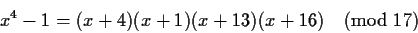In simple terms, for every multiplication/division that we have in the expression we have to multiply n by 2 (add one bit to its length). This bounds the length of n to the size of the expression being tested.

Remember that this heuristic makes mistakes only if Sn(e)=0. If, then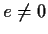.

Our approach for computing (better) heuristics will be to select n from some properties or features of the expression. In this way we can make n large enough to satisfy our probability constraints and satisfy some other constraints that will be seen later.Next: Rational expressions Up: Heuristic equivalence testing and Previous: Heuristic equivalence testing and
Gaston Gonnet
1999-07-04